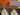## How to Determine Your Roof Slopecaption

The real difference between roof slope and roof pitch

There’s a lot of debate around the correct definition of a roof slope and a roof pitch. Today we are going to understand the key difference between the two and how to determine the slope of a roof.

## Slope Vs Pitch

The slope of a roof can be defined as the measurement of the rise of the roof to the base of the roof from the center which is essentially called the ‘run of the roof’. This distance is measured from the center of the ridge to one of the ends of the roof and the slope of the roof is the ratio between the height and the length of the roof from the center.Assuming the roof to be a right triangle, the slope of the roof will be measured as the ratio between BD and DC. The distance from the center of the ridge to the side(DC) in this case will be 4.25 meters and the height(BD) is approximately 4.24 meters. Therefore the slope of the roof will be the ratio between the two distances.

The pitch of the roof, on the other hand, is the measurement between the height of the roof(BD) and the entire span of the base of the roof (AC) and is represented in a fraction instead of a ratio.

## Measuring the slope

There are a lot of different ways to measure the slope of a roof. One of the best ways to do so is by measuring from the underside with the help of the rafter. Homes with attics have a rafter which can be accessed in order to measure the slope. As rafters provide an even surface for measurement, the results are proven to be the most accurate in terms of determining the exact ratio of the slope.

The process requires you to place a level against the underside of the rafter. Make sure that the bubble in the level is at the center of the two lines and that the level is always in position. Next, find the 12-inch mark from the level on the rafter. Once you find the mark, measure the distance of the roof right above it and bingo! Just jot down the measurements and you have the slope of your roof. The measurements don’t need to be broken down and can be presented in a simple ratio. Suppose the height of the ridge from the 12-inch mark is 5 inches, then the slope would simply be 5:12.

However, in some cases, the rafter is not accessible in order to measure the slop and a good alternative is to climb your roof and measure the slope from the roof of the surface. It is very important to make sure that the roof is accessible and safe to walk on. The process of measuring the slope is not difficult but one just needs to be careful about working on the roof.

The first step is to make a mark on 12 inches on the level and make sure that the bubble is at the center. The next step is to use a measuring tape and determine the distance of the slope. You can take a look at this video to observe how it’s done.

Both these methods are proven to derive accurate measurements of the slope. As an adjuster, if you’re taking measurements while being on top of a roof, you need to be careful when moving around the roof in order to prevent yourself from falling. It is best to assess the condition of the roof before climbing it to determine whether the roof is accessible or not. The tools required for this job are only a level and a measuring tape that can be easily carried.

Measuring roof slope can be helpful for estimation purposes and can also help determine the roofing material that will be required for various kinds of roofing jobs. Having a sound knowledge of the dimensions of the roof along with these methods will help you to become more accurate at determining the roof slope.